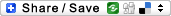# Difference between revisions of "Sandbox"Note: ASCIIMath has been replaced by Mimetex

ASCIIMath:

This is a test equation <am>int_-1^1 (a+b)/(x+y)</am>

<am>[[a,b],[c,d]]</am>

<am>sqrt sqrt root3 x</am>

<am>alpha beta chi Delta delta del</am>

Mimetex: